# Frustum of a Cone

• Last Updated : 24 Nov, 2021

In our daily life, we come across a lot of objects where we need to find their volume and surface areas. For example, we might have to calculate how much water a tank can hold. For this, we need to know the shape of the tank and furthermore, we need to know how to calculate its volume. Sometimes some solids are a combination of different standard shapes.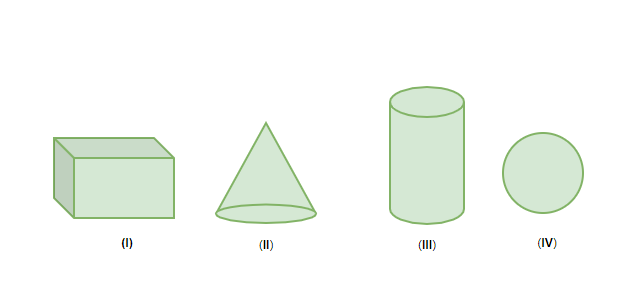The figure above shows some basic solid shapes. Objects are usually made up of a combination of these shapes. But sometimes we can make another shape out of a solid by removing a portion of it. For example, Frustum. In this shape, we are interested in removing a portion of a cone. Let’s study the frustum in detail.

### Frustum

Let’s assume a right circular cone and remove a portion out of it. Let’s cut the cone with a plane parallel to the base.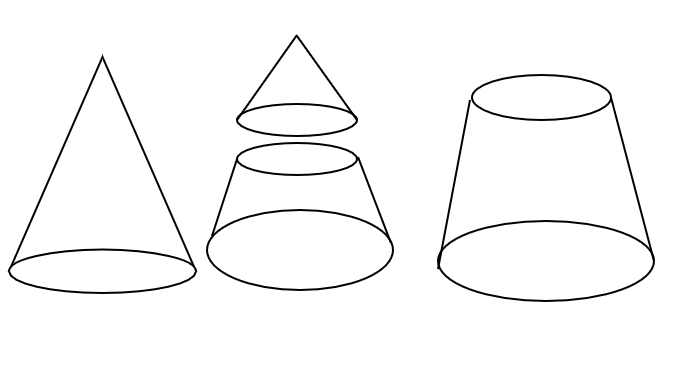The shape which remains after cutting off the small upper cone is called a frustum. We all have seen this shape a lot in our daily lives, our drinking glasses are in this shape. So, the frustum can be defined as,

If a right circular cone is cut off by a plane parallel to it’s base, the shape of the portion between the cutting plane and the base plane is called frustum of that cone.

Let’s look at the volume and surface area of this shape.

### Volume of a Frustum

The definition of frustum given above states that frustum is a sliced part of a cone. So, to calculate the volume of the frustum we just need to calculate the difference between the volume of the larger and smaller cone.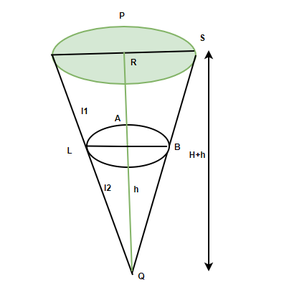In the figure given above let’s assume,

1. The total height of the cone to be “H + h”
2. Total slant height to be “l1 + l2”
3. The radius of bigger cone = R
4. The radius of sliced cone = r

The total volume the cone V1The Volume of the smaller cone which was removed V2So now let’s calculate the volume of the frustum (V)

V = V1 – V2

=–=Now using the formula of tangent function in trigonometry we get,Plugging in this value of H+h in the formula for Volume.

V =Using the similar triangle’s property again, we will find out the value of h.Substituting this value of h in the formula, we get### Surface Area of the Frustum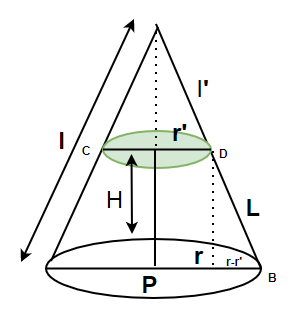Similar to Volume, the surface area will also be equal to the difference between the surface areas of the bigger cone and the smaller cone.

The curved surface area of bigger cone = π Rl

The curved surface area of smaller cone = π rl’

Difference between surface areas = π (Rl – rl’)

In the figure given above, triangles OAB and OCD are similar. So,Let’s calculate circumferences of the bases,

C = 2π r and C’ = 2π r’

So, the Total curved surface area of the frustum A,Here, L is the slant height given by, L =The total surface area of frustum = Total curved surface area of the frustum A + Area of both the bases

Let’s see some problems related to these formulas we just derived.

### Sample Problems

Question 1: Find out the Volume of a frustum of a cone which is 20cm high and the radii for both the bases are 5cm and 8cm.

Solution:

Using the formula studied above,We are given, H = 20cm, r = 5cm and R = 8cm. Plugging these values in the equation.Question 2: Find out the surface area and total surface area of a frustum of a cone which is 10cm high and the radii for both the bases are 4cm and 8cm.

Solution:

We know the formula for surface area and total surface area of the frustum. We need to plug in the required values.

Curved Surface area of the frustum =Where LGiven, H = 10cm, r = 4cm and r’ = 8cm

Calculating the value of L, L =Curved Surface area of the frustum =Total Surface Area = Curved surface are of the frustum + Area of both the bases

=Question 3: Let’s say we have an open metal bucket whose height is 50cm and the radii of the bases are 10cm and 20cm. Find the area of the metallic sheet used to make the bucket.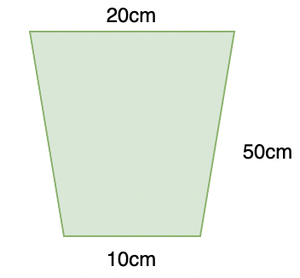Solution:

Bucket is in the form of frustum which closed from the bottom. We need to calculate the total surface area of this frustum.

Given : H = 50cm, r1 = 10cm and r2 = 20cm.

Curved surface area of the frustum S =Let’s calculate L first,Putting this value in the surface area of the formula,This is the curved surface. To find the total area (A) of the metal sheet used,

A = S + Area of the bottomQuestion 4: Find out the expression of the volume for a frustum if its height is 5y cm, radii are y and 2y.

Solution:

Using the formula studied above,We are given, H = 5y, r = y and R = 2y. Plugging these values in the equation.My Personal Notes arrow_drop_up